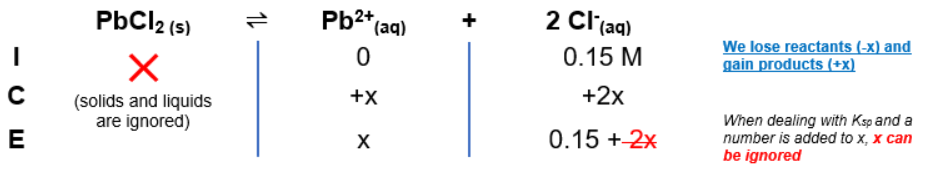# Problem: What is the solubility (in M) of PbCl2 in a 0.15 M solution of HCl? The Ksp of PbCl2 is 1.6 x 10-5.

###### FREE Expert Solution

To solve for molar solubility of PbCl2 in 0.15 M HCl

Recall that Ksp is an equilibrium constant related to slightly soluble ionic compounds

The dissociation of slightly solublePbCl2 in solution is as follows:

PbCl2(s)  Pb2+(aq) + 2Cl(aq)

We can construct an ICE table for this dissociation. Remember that solids are ignored in the ICE table and Ksp expression.85% (117 ratings)###### Problem Details

What is the solubility (in M) of PbCl2 in a 0.15 M solution of HCl? The Ksp of PbCl2 is 1.6 x 10-5.